# ML basics : Feature Selection (Part 2)

Welcome back.

The background story of what exactly are we doing here is in here.

Now, moving forward.

# Backward Elimination

We will be selecting features based on backward elimination method.

What exactly is backward elimination? This is a method to keep only those features which are significant to the dataset i.e. considering those features do considerable amount of change to the dependent variable.

Here is the way this algorithm works :

1. Select a significance level.
2. Fit the model with all features.
3. Check the p-values of different features with summary() function.
4. If p-value is higher than significance level, remove the feature.
5. Repeat step 2 to 4 with the reduced features till only the features having p-values ≤ significance level remain.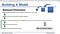Basically! courtesy- Udemy

# Significance level and p-value

So, significance level is the amount of change a feature will affect towards the final output i.e. how important is this feature and how much it affects the final output. Generally, we take 5%/0.05 significance level by default.

p-value refers to the hypothesis of the significance level.

Let’s say you have a friend who says that a feature is absolutely of no use. (that is called as null hypothesis). The higher the p-value’s value is, the more he is correct and vice versa.

p-value goes from 0 to 1.

So say column 1 has p-value of 0.994, null hypothesis is true i.e. this column does not provide any noticeable change to the output and can be easily removed without consequences.

Now, column 2 has a p-value of 0.001, null hypothesis is false i.e. is provides very significant change to the output.

Where do we draw the line of something being significant or not? Well that is where significance level comes in.

In our case we won’t consider any p-values over 0.05.

Code:

Code explaination :

The above code block is simply training a dataset in linear regression. Added b0x0 as that will be the first feature column. Since,

y = mx + c or

y = b0x0 + b1x1

Basically it is a column of ones. Next step is to import statsmodel.formula.api module as sm.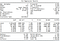Result of the summary() function.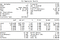Second summary()

Removing the highest p-value(x2 or 2nd column) and rewriting the code.Third summary()

Removing the highest p-value(x1 or first column) and rewriting the code.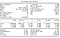Fourth Summary()

Removing the highest p-value(x3 or 4th column) and rewriting the code.

Now, we have a decision to make, we can see that x2 or 5th column has a p-value of 0.06 or 6%. It is quite close to the significance level of 5% so we are not completely sure if removing it will be a good idea.

When in doubt, we will check the adjusted R-square values for higher accuracy.

Current Adj. r-squared value : 94.8%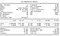Fifth/Final Summary()

Removing the highest p-value(x2 or 5th column) and rewriting the code.

New Adj. r-squared value : 94.5%

We can see our accuracy decreased thus we will keep the previous columns. Thus the relevant columns for us in this dataset are 3rd and 5th column and thus we can drop all the other columns without having any decrease in model accuracy.

Well that is it for now.

Bye.